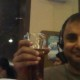# DecisionStats Summer School in Delhi 2015 #rstats

This summer get a foothold in the world of data science. These are in classroom trainings for Delhi India and all prices are in INR only.

If you are interested apply here-

bit.ly/decisionstatsSUMMER SCHOOL 2015

• Bring your own device. Hardware – with >2GB RAM and >20 GB Hard Disk Free
• Eligibility Criterion – People Interested in a career as a data scientist. No prior skills are required but statistics and programming can help.
• 1 class is of 2.5 hours followed by a break of 1 hour . Each Day has two classes per batch

Course Details

 15 – 16 June 17- 18 June 19 June – 22 ,23,24 June 25 -26 June Course Name Introduction to Data Science Introduction to Analytics using Python Introduction to Analytics using R SAS Language Fundamentals Hours 10 10 20 10 Classes 4 4 8 4 Days 2 2 4 2 Cost 8000 10000 12000 15000 25000

Taking all four courses gives you a saving of 80% with 50 hours total class time.

Instructor will teach in person and open for clearing doubts on the spot.

Course Outline

 Basics of Data Science Introduction to Python Introduction to R Introduction to Interface Basics of Analytics Introduction to iPython Introduction to R Studio Introduction to SAS language LTV Analysis Introduction to Pandas Introduction to R Data Step LTV Analysis Quiz Introduction to iPython Notebook Introduction to Rattle Proc Print RFM Analysis IDE- IDLE and Spyder Deducer Proc Means and Proc Freq RFM Analysis Quiz Python 1 Quiz R Quiz 1 SAS Quiz 1 Basic Stats Data Input Data Input Proc Univariate Introduction to Modeling Data Analysis Data Analysis Do loops Data Summarization Data Summarization Proc sgplot Introduction to Google Analytics Data Visualization Data Visualization Proc SQL Blogging Data Output Data Output SAS Macro Language Web Analytics Quiz Ipython 2 Quiz R Quiz 2 menu driven options data.table ODS Output ggplot sports analytics SAS Quiz 2 regression model data mining R Quiz 3 social network analysis text mining time series forecasting Using apis association analysis R Quiz 4 RODBC sqldf spatial analytics RMarkDown Using Github R Quiz 5

If you are interested apply here-

bit.ly/decisionstats